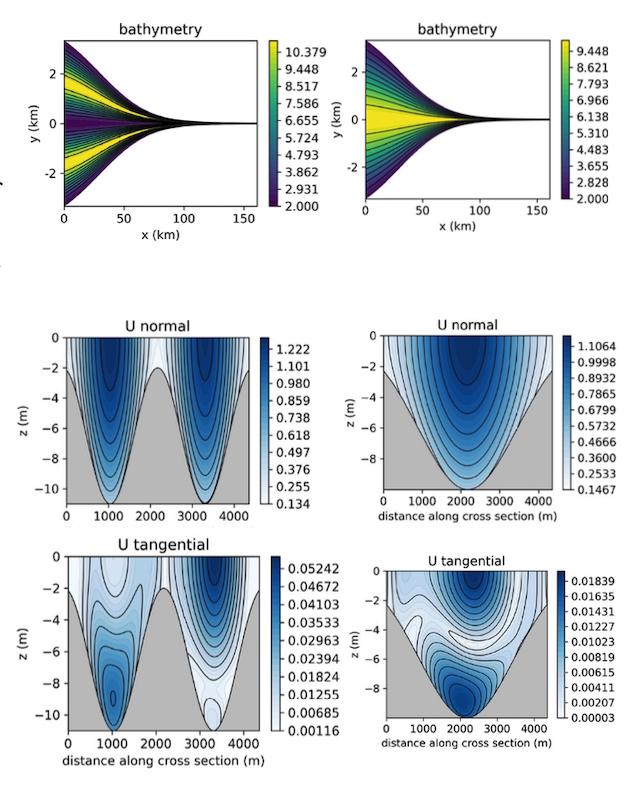M.P. Rozendaal1*, Y.M. Dijkstra1, H.M. Schuttelaars1,

1 Delft Institute of Applied Mathematics, Delft University of Technology;

*corresponding author: This email address is being protected from spambots. You need JavaScript enabled to view it.

Introduction

The water motion in tidally-dominated estuaries is greatly influenced by the geometry and bathymetry. To study this interaction, a 3D model is required, where both the longitudinal and lateral processes are resolved. Two types of process-based models are typically employed to get insight into these dynamics. High complexity 3D numerical models, such as TELEMAC and Delft3D, and idealised 3D models, such as the model of Kumar (2018). The latter models are explicitly developed to gain insight in the dominant physical processes and to systematically analyse the influence of parameters by performing parameter sensitivity studies. Up till now, 3D idealised models were restricted to idealised geometries and simple bathymetries. To systematically analyse the influence of complex geometries and bathymetries on the water motion, we developed a new idealised model, iFlow3D.

Methods

The iFlow3D model solves the three-dimensional shallow water equations using a perturbative approach. The resulting equations are solved in the frequency domain, allowing for the direct computation and identification of prescribed tidal constituents, e.g., M0, M2, M4 etc. The governing equations can be decoupled in the vertical dimension and the resulting 1DV problem can be solved analytically. Thus the 3D problem is reduced to a 2DH problem. To obtain fast and numerically accurate solutions, the 2DH problem is solved using a high-order finite element method (spectral element method with modal basis functions) with curved elements, which is essential to obtain accurate first- and second-order numerical derivatives in curved geometries. The bathymetry can be prescribed in terms of along- and across- channel coordinates, allowing for a systematic approach to study the influence of the bathymetry and geometry on the water motion.

Results

To illustrate the strength and versatility of the iFlow3D model, a sensitivity study is performed in which the bathymetry is slowly varied. An idealised estuary inspired by the characteristics of the Scheldt estuary is considered. In the converging geometry, a two channel bathymetry is morphed into a single channel bathymetry. The effects of this transition on the water motion is illustrated in Fig. 1. In the presentation, I will systematically study the generation mechanisms of the residual and tidal flows under changing geometry and bathymetry.Figure 1: Example of an idealised estuary with a two channel and one channel bathymetry. At km 25, a cross section is taken. The amplitude of the M2 velocity along channel (normal) and across channel (tangential) are shown.

References

M.Kumar. Three-Dimensional Model For Estuarine Turbidity Maxima In Tidally Dominated Estuaries. PhD thesis, Delft University of Technology, 2018.